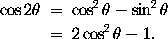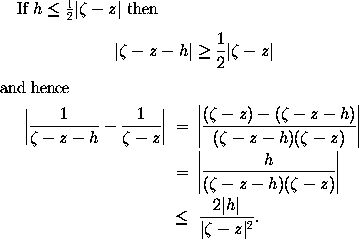Multiline Formulae in LaTeX

Consider the problem of typesetting the formulaIt is necessary to ensure that the = signs are aligned with one another. In LaTeX, such a formula is typeset using the eqnarray* environment. The above example was obtained by typing the lines
\begin{eqnarray*}
\cos 2\theta & = & \cos^2 \theta - \sin^2 \theta \\
& = & 2 \cos^2 \theta - 1.
\end{eqnarray*}
Note the use of the special character & as an alignment tab. When the formula is typeset, the part of the second line of the formula beginning with an occurrence of & will be placed immediately beneath that part of the first line of the formula which begins with the corresponding occurrence of &. Also \\ is used to separate the lines of the formula.

Although we have placed corresponding occurrences of & beneath one another in the above example, it is not necessary to do this in the input file. It was done in the above example merely to improve the appearance (and readability) of the input file.

The more complicated examplewas obtained by typing
If $h \leq \frac{1}{2} |\zeta - z|$ then
$|\zeta - z - h| \geq \frac{1}{2} |\zeta - z|$
and hence
\begin{eqnarray*}
\left| \frac{1}{\zeta - z - h} - \frac{1}{\zeta - z} \right|
& = & \left|
\frac{(\zeta - z) - (\zeta - z - h)}{(\zeta - z - h)(\zeta - z)}
\right| \\  & = &
\left| \frac{h}{(\zeta - z - h)(\zeta - z)} \right| \\
& \leq & \frac{2 |h|}{|\zeta - z|^2}.
\end{eqnarray*}

The asterisk in eqnarray* is put there to suppress the automatic equation numbering produced by LaTeX. If you wish for an automatically numbered multiline formula, you should use \begin{eqnarray} and \end{eqnarray}.